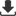ISSN 2415-1297 (Online)   ISSN 2415-1300 (Print)Volume : 27 Issue : 3 Year : 2019Current Issue Ahead of Print Most Accessed Articles Archive Online SubmissionCopyright Transfer FormA New Technique (Holotransformation) for Evaluation of A Forced Convection Equation from Experimental Data [Med J Islamic World Acad Sci]
Med J Islamic World Acad Sci. 1989; 2(3): 155-159

## A New Technique (Holotransformation) for Evaluation of A Forced Convection Equation from Experimental Data

Edip Büyükkoca
From the Chemical Engineering Department, Engineering Faculty of Yildiz University Abide-i Hürriyet Cad., Sisli-Istanbul, Turkiye.

In the mathematical modelling of engineering studies, when we want to make an evaluation on the experimental data we meet a significant problem that is the elimination of uncertainties in observed data. Its nature and magnitude is associated with both measurement error and our models of the systems of chemical, biological and environmental. In this work, a new technique (Holotransformation) is proposed for evaluation of a forced convection equation from experimental data. It has been demonstrated and compared by an example which is carried out "Proces Heat Transfer", D.Q. Kern, Mc Graw-Hill. P. 56-53 (1950) (6). By the Holotransformation, the problem is reflected from Eucludian space into a non-Eucludian space by a suitable transformation matrix. The solution of problem is always made in non-Eucludian space by the conventional techniques. A back reflection (Holotransformation) gives Euclidian space's solution which is the solution of original problem. The application of Holotransformation on the solution of normal equations of linear least squares problem shows that it gives an ability to find out more proper and simplest mathematical formula for observed data.

Keywords: Holotransformation, forced convection equation, mathematical modelling

Edip Büyükkoca. A New Technique (Holotransformation) for Evaluation of A Forced Convection Equation from Experimental Data. Med J Islamic World Acad Sci. 1989; 2(3): 155-159

Corresponding Author: Edip Büyükkoca, Türkiye

 TOOLSFull Text PDFPrintDownload citationRISEndNoteBibTexMedlarsProciteReference ManagerShare with emailShareSend email to authorSimilar articlesPubMedGoogle Scholar